### Day 27 - Implicit Differentiation - 02.17.15

Update

Bell Ringer

Calculate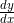for the following:
1.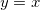1. x

2. y

3. 1

4. 0

5. none of the above

2.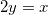1.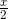2.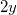3. 2

4. 0

5. none of the above

3.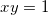1.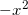2.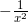3.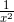4.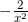5. none of the above

4.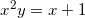1.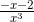2.3.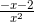4.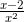5. none of the above

5.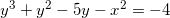1.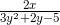2.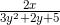3.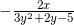4.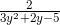5. none of the above

Review

Lesson
• Implicit Differentiation

Exit Ticket
• Posted on the board at the end of the block
Lesson Objectives
• How is implicit differentiation used to solve problems?

#### In-Class Help Requests

Standard(s)
• APC.5
• Investigate derivatives presented in graphic, numerical, and analytic contexts and the relationship between continuity and differentiability.
• The derivative will be defined as the limit of the difference quotient and interpreted as an instantaneous rate of change.
• APC.6
• ​The student will investigate the derivative at a point on a curve.
• Includes:
• finding the slope of a curve at a point, including points at which the tangent is vertical and points at which there are no tangents
• using local linear approximation to find the slope of a tangent line to a curve at the point
• ​defining instantaneous rate of change as the limit of average rate of change
• approximating rate of change from graphs and tables of values.
• APC.7
• Analyze the derivative of a function as a function in itself.
• Includes:
• comparing corresponding characteristics of the graphs of f, f', and f''
• ​defining the relationship between the increasing and decreasing behavior of f and the sign of f'
• ​translating verbal descriptions into equations involving derivatives and vice versa
• defining the relationship between the concavity of f and the sign of f "
• APC.9
• Apply formulas to find derivatives.
• Includes:
• derivatives of algebraic and trigonometric functions
• derivations of sums, products, quotients, inverses, and composites (chain rule) of elementary functions
• derivatives of implicitly defined functions
• higher order derivatives of algebraic and trigonometric functions

Past Checkpoints
• Derivative
• Derivative Rules
• Higher Order Derivatives
• Rates of Change
• Position Function
• Velocity Function
• Acceleration Function
• Product Rule
• Quotient Rule
• Chain Rule
• Implicit Differentiation
• Checkpoints
• A - page 146 #6
• B - page 146 #10
• C - page 146 #26
• D - page 146 #30
• E - page 147 #48
• F - page 147 #52
• G - page 147 #66
• H - page 148 #74
• I - page 148 #78
• Related Rates
• Checkpoints
• A - page 154 #2
• B - page 154 #16
• C - page 154 #18
• D - page 154 #20
• E - page 154 #14